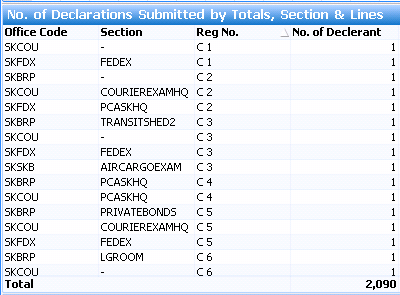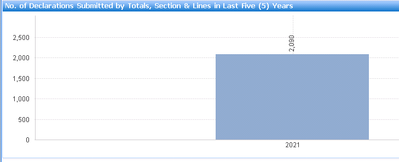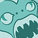Announcements
Applications are open for the 2024 Qlik Luminary Program. Apply by December 15 here.
cancel
Showing results for
Did you mean:Specialist

## Expression calculation in bar chart with group by

Hello Team,

I have a straight table where I put a expression like
No. of Decleration = Count( distinct reg_num)

Note: I have  selected the radio button sum of rows in the expression tab of chart property.

it is showing result =2090

Example:I have to show the same result in bar chart. I am using the same expression like          = Count( distinct reg_num)

But getting wrong data in the chart as it is not applying group by on dimensions office_code and section.How to apply group by on chart level and get the same total amount as in straight table?

I used aggr function but did not get result.

aggr(distinct reg_num,section,off_cod)

Thanks,

Narender

2 Solutions

Accepted SolutionsMVP

@narender123  try below

=sum(aggr(Count( distinct reg_num) , section,off_cod))Specialist
Author

It is working fine now.

=sum({<Year={'>=\$(=max(Year) -4) '}>} aggr(count ({<Year={'>=\$(=max(Year) -4) '}>}distinct reg_num) , section, off_cod, year))

6 RepliesMVP

@narender123  try below

=sum(aggr(Count( distinct reg_num) , section,off_cod))Specialist
Author

Hi Kush,

Point 1: This works fine for the 1 year like

=sum(aggr(Count({<Year={\$(=max(Year))}>} distinct reg_num) , section,off_cod))Point 2: But I need to show last five count. so it is not showing the last 5 years, also showing the wrong data for 2021.

=sum(aggr(Count({<Year={'>=\$(=max(Year)-4)'}>} distinct reg_num) , section,off_cod))Point 3: My last 5 year count works when I don't use aggr but getting wrong data count

=Count({<Year={'>=\$(=max(Year)-4)'}>} distinct reg_num)Please tell me, how to show last 5 year count with aggr.

Thanks,Contributor

I really appreciated your post for the new user I really like this .

ACES ETMMVP

HI

Might be, try like below

=sum(aggr(Count( {<Year={'>=\$(=max(Year)-4)'}>} distinct reg_num) , Year, section,off_cod))

Thanks & Regards, Mayil Vahanan RSpecialist
Author

Hi   ,

But it shows only 2021 year count which is right count for 2021 but it need to show 2017,2018,20199,2020 count also.Specialist
Author

It is working fine now.

=sum({<Year={'>=\$(=max(Year) -4) '}>} aggr(count ({<Year={'>=\$(=max(Year) -4) '}>}distinct reg_num) , section, off_cod, year))